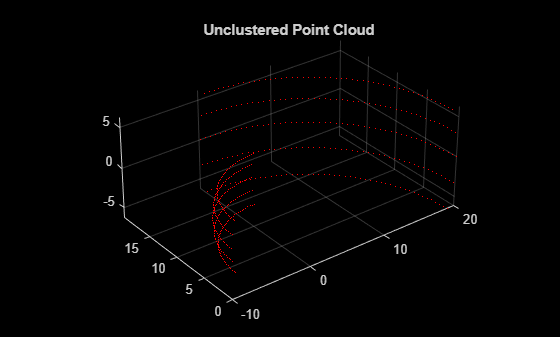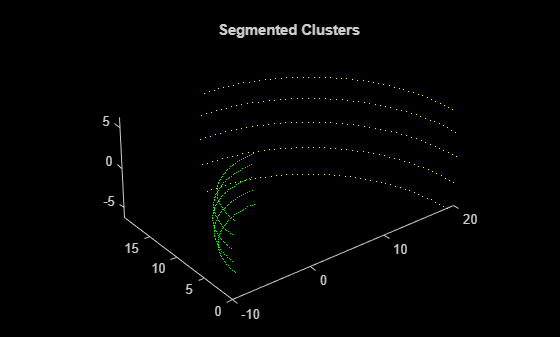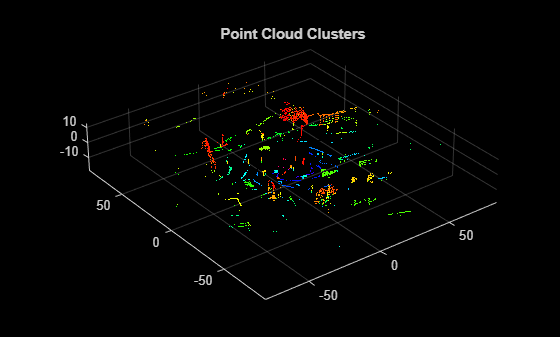# segmentLidarData

Segment organized 3-D range data into clusters

## Syntax

``labels = segmentLidarData(ptCloud,distThreshold)``
``labels = segmentLidarData(ptCloud,distThreshold,angleThreshold)``
``[labels,numClusters] = segmentLidarData(___)``

## Description

example

````labels = segmentLidarData(ptCloud,distThreshold)` segments organized 3-D range data, `ptCloud`, into clusters. The function assigns an integer cluster label to each point in the point cloud, and returns the cluster label of all points in `labels`. Two neighboring points are grouped into the same cluster if their Euclidean distance is less than `distThreshold`.```

example

````labels = segmentLidarData(ptCloud,distThreshold,angleThreshold)` segments the data using an additional constraint based on the angle between the sensor and two neighboring points. The line passing through the sensor and one point forms the first side of the angle. The line passing through the two neighboring points forms the second side of the angle. The two points are grouped into the same cluster if the angle is greater than `angleThreshold`.```
````[labels,numClusters] = segmentLidarData(___)` also returns the number of clusters.```

## Examples

collapse all

Create organized synthetic lidar data containing two objects.

```ldr = zeros(5,100); ldr(:,1:50) = 10; ldr(:,51:end) = 20; pitch = linspace(-18,18,5); pitch = repmat(pitch',1,100); yaw = linspace(-90,90,100); yaw = repmat(yaw,5,1); ```

Convert to Cartesian coordinates.

```X = ldr .* cosd(pitch) .* sind(yaw); Y = ldr .* cosd(pitch) .* cosd(yaw); Z = ldr .* sind(pitch); pc = pointCloud(cat(3,X,Y,Z)); figure pcshow(pc.Location,'r') title('Unclustered Point Cloud')```Set the threshold.

`distThreshold = 5;`

Segment the lidar data.

`labels = segmentLidarData(pc,distThreshold);`

Plot the results.

```figure hold on title('Segmented Clusters') pc1 = select(pc,find(labels == 1)); pcshow(pc1.Location,'g') pc2 = select(pc,find(labels == 2)); pcshow(pc2.Location,'y')```Set up the PCAP file reader.

`veloReader = velodyneFileReader('lidarData_ConstructionRoad.pcap','HDL32E');`

Wait for 0.3 seconds from the beginning of the file, then read the point cloud from the next frame.

```veloReader.CurrentTime = veloReader.StartTime + seconds(0.3); ptCloud = readFrame(veloReader);```

Segment and remove the ground plane.

```groundPtsIdx = segmentGroundFromLidarData(ptCloud); ptCloudWithoutGround = select(ptCloud,~groundPtsIdx,'OutputSize','full');```

Cluster the remaining points. Distance is in meters.

```distThreshold = 0.5; [labels,numClusters] = segmentLidarData(ptCloudWithoutGround,distThreshold);```

```numClusters = numClusters+1; labels(groundPtsIdx) = numClusters;```

Plot the labeled results. Display the ground plane in black.

```labelColorIndex = labels+1; pcshow(ptCloud.Location,labelColorIndex) colormap([hsv(numClusters);[0 0 0]]) title('Point Cloud Clusters')```## Input Arguments

collapse all

Point cloud, specified as a `pointCloud` object. `ptCloud` is an organized point cloud that stores point coordinates in an M-by-N-by-3 matrix. The points must be organized by pitch and yaw angles in a sequential scanning order, as is typically returned by laser range finders or `velodyneFileReader`.

Distance threshold in world units, specified as a nonnegative scalar. To reduce the number of output clusters, increase the value of `distThreshold`.

Data Types: `single` | `double`

Angle threshold in degrees, specified as a scalar in the range [0, 180]. To reduce the number output clusters, decrease the value of `angleThreshold`.

Data Types: `single` | `double`

## Output Arguments

collapse all

Cluster labels of all points in the point cloud `ptCloud`, returned as an M-by-N matrix of integers. Each valid point in `ptCloud` belongs to a cluster. All points in a cluster are assigned the same integer cluster label, ranging from `1` to `numClusters`. Invalid points, such as points with `Inf` or `NaN` coordinates, are assigned the label `0`.

Number of clusters of valid points, returned as a nonnegative integer. The number of clusters does not include the cluster corresponding to invalid points.

 Bogoslavskyi, I. “Efficient Online Segmentation for Sparse 3D Laser Scans.” Journal of Photogrammetry, Remote Sensing and Geoinformation Science. Vol. 85, Issue 1, 2017, pp. 41–52.